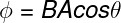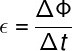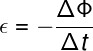Electricity & Megnetism

# Lenz’s Law

Lenz’s law states that, when there is a change in magnetic flux over a closed conductor, a magnetic field will appear in that conductor that will oppose such change. This law is a direct consequence of a principle in physics known as conservation of energy and complements Faraday’s law of induction .

## Introduction to Lenz’s Law

Lenz’s law was established by Russian physicist Heinrich Lenz and contributed another law that already existed: Faraday’s law, also known as the law of electromagnetic induction. Lenz’s law gave a new meaning to Faraday’s law by taking into account the principle of conservation of energy.

The law of electromagnetic induction had been discovered by Michael Faraday in 1831. According to this law, a variable magnetic flux is capable of producing an induced electric current inside a closed conductor , such as wires, metallic plates, turns or metallic coils.

Lenz’s law considered that the direction of the electric current induced in the conductors was such that the magnetic field generated by this current should oppose the variation of the magnetic flux .

Lenz’s conclusion was based on the idea that it is not possible to produce electrical energy at will, in order to do so, a certain amount of energy would need to be consumed . This energy consumed, in turn, is equivalent to the work required to bring a magnet closer or farther away from a conducting loop, since the relative motion between magnet and loop gives rise to a force that opposes the motion.

## magnetic flux

The magnetic flux is a scalar quantity whose unit of measure is the weber (Wb) and which can be calculated by the product of the intensity of the magnetic field B, the area being traversed by the field lines and the cosine of the angle formed between the vector B and the normal line to the surface of area A, check:Φ – magnetic flux (T.m² or Wb)

B – magnetic field (T)

A – area (m²)

θ – angle between B and the normal of A (º)

## Induced electromotive force

According to Faraday’s law, the induced electromotive force is the electric potential , in volts, produced by changing a magnetic flux with respect to time , as shown in the following formula:ΔΦ – variation of magnetic flux (Wb)

With contributions from Lenz’s law, a mathematical sign was added to the previous law. This sign expresses that the polarity of the induced electromotive force is contrary to the variation of the magnetic flux:The following figure shows the direction of the magnetic field induced in the coils or turns according to the direction of movement ( v ) and variation of the external magnetic field ( ΔB ), note:

## Applications of Lenz’s Law

Check out some of the main technological applications that make direct or indirect use of Lenz’s law:

• Electric generators  One of the main applications of Lenz’s law concerns the direction of the electric current that is produced in electricity generators. Since generators produce electrical energy, according to the rotational movement of a set of magnets with respect to a coil, the direction of the induced electrical current varies periodically. This current is known as alternating current and is the electrical current that reaches homes through high voltage wires.
• Transformers – Electrical transformers are also direct applications of Lenz’s law. These devices are used to increase or decrease the electrical voltage of the transmission lines, for that, they make use of the variation of the magnetic flux between turns with different numbers of windings.
• Metal detector – Metal detectors produce an oscillating magnetic field. When a metal is passed through this magnetic field, it gives rise to a changing and opposing magnetic field that can be easily detected.
• Magnetic brake  Another example of Lenz’s law application is the magnetic brake, in which a powerful magnet is brought close to an aluminum disk. When the disk is rotating, there is a change in the magnetic flux over it, in this way, a magnetic force arises, opposing such change, in this way, the disk loses speed.

## Exercises on Lenz’s Law

Question 1 – (Enem) Dynamos are electric power generators used in bicycles to light a small light bulb. For this, it is necessary that the moving part is in contact with the bicycle tire and, when it starts to move, electrical energy is generated to light the lamp. Inside this generator are a magnet and a coil.

Available at: http://www.if.usp.br. Accessed on: 1 May 2010

The working principle of this equipment is explained by the fact that:

A) Electric current in the closed circuit generates a magnetic field in this region.

B) coil immersed in the magnetic field in closed circuit generates an electric current.

C) coil in friction with the magnetic field in the closed circuit generates an electric current.

D) Electric current is generated in a closed circuit because of the presence of the magnetic field.

E) Electric current is generated in a closed circuit when the magnetic field changes.

Template: Letter E

resolution

The variation of the flux of a magnetic field through a closed conductor produces an induced electromotive force , therefore, the correct alternative is the letter D .

Question 2 – (Fuvest) A magnet is brought close to a metallic ring fixed on an insulating support, as shown in the figure. The movement of the magnet, towards the ring,

A) has no effect on the ring.

B) produces alternating current in the ring.

C) causes the south pole of the magnet to become the north pole and vice versa.

D) produces electric current in the ring, causing a force of attraction between ring and magnet.

E) produces electric current in the ring, causing a repulsive force between ring and magnet.

Template: Letter E

resolution

According to Lenz’s law, the approximation between the magnet and the loop causes a magnetic field opposite to the variation of magnetic flux to appear, producing a repulsive force between the magnet and the loop, so the correct alternative is the letter E .